# NCERT Solutions for Class 8 Maths Chapter 1 Rational Numbers InText Questions

These NCERT Solutions for Class 8 Maths Chapter 1 Rational Numbers InText Questions and Answers are prepared by our highly skilled subject experts.

## NCERT Solutions for Class 8 Maths Chapter 1 Rational Numbers InText Questions

Try These (Page No. 4)

Question 1.
Fill in the blanks in the following table.Solution:
Using the closure property over addition, subtraction, multiplication, and division for rational numbers, integers, whole numbers, and natural numbers, we have: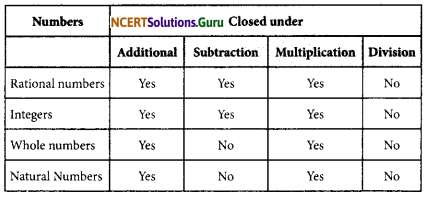Try These (Page No. 6)

Question 2.
Complete the following table: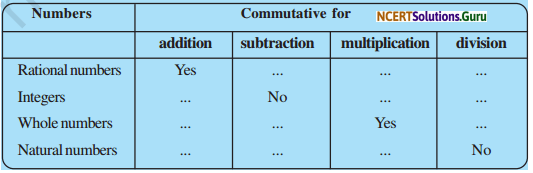Solution: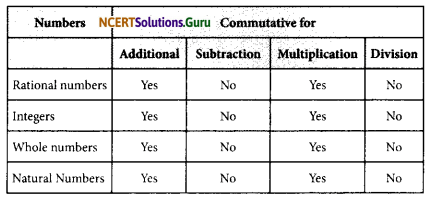Try These (Page No. 9)

Question 3.
Complete the following table:Solution: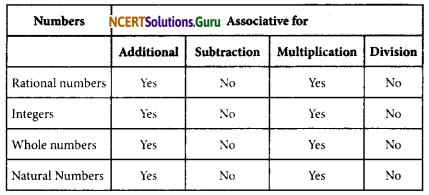Try These (Page No. 13)

Question 4.
Find using distributivity.Solution:Try These (Page No. 17)

Question 5.
Write the rational number for each point labelled with a letter.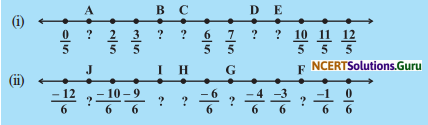Solution:
(i) Here, the rational number for the point A is $$\frac{1}{5}$$.
The rational number for the point B is $$\frac{4}{5}$$.
The rational number for the point C is $$\frac{5}{5}$$.
The rational number for the point D is $$\frac{8}{5}$$.
The rational number for the point E is $$\frac{9}{5}$$.(ii) The rational number for:
The point F is $$\frac{-2}{6}$$ or $$\frac{-1}{3}$$.
The point G is $$\frac{-5}{6}$$.
The point H is $$\frac{-7}{6}$$.
The point I is $$\frac{-8}{6}$$ or $$\frac{-4}{3}$$.
The point J is $$\frac{-11}{6}$$.

error: Content is protected !!